##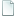Topic: [code] Gravity Simulation wth Demo (Read 4683 times)previous topic - next topic

0 Members and 1 Guest are viewing this topic.
• Flying Jester
•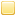• TurboSphere Developer
[code] Gravity Simulation wth Demo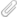##### December 19, 2013, 05:49:37 am
A quick little demo I made for modelling gravity.• DaVince
••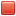• Used Sphere for, like, half my life
Re: Gravity Simulation
##### Reply #1 – December 19, 2013, 04:08:57 pm
Oh, an spk. It's been a while since anyone actually used that.
(You should totally add support for that in TS, if it doesn't already. Maybe even as a .tspk extension to indicate it's a TS game.)

So, tried it. It's interesting, but when I run the demo, I get a circle constantly circling another circle. Don't have an SPK unpacker handy at the moment; is there anything special you can demonstrate with it? Like how the arch will change depending on gravity pull, or stuff?

••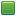• Senior Staff
• Wise Warrior
Re: Gravity Simulation
##### Reply #2 – December 19, 2013, 05:10:11 pm

Oh, an spk. It's been a while since anyone actually used that.
(You should totally add support for that in TS, if it doesn't already. Maybe even as a .tspk extension to indicate it's a TS game.)

I should add that to SFML version too! I think it's just a zip file, basically. But 7zip won't unpack it, so I guess it's missing some header stuff.
If you use code to help you code you can use less code to code. Also, I have approximate knowledge of many things.

Sphere-sfml here
Sphere Studio editor here

• DaVince
••• Used Sphere for, like, half my life
Re: Gravity Simulation##### Reply #3 – December 19, 2013, 05:17:29 pm
Yeah, it's something zlib-based with a custom header, I believe. Have this Sphere-based SPK unpacker! (Don't remember who wrote it, but, well, I had it, so...)

••• Senior Staff
• Wise Warrior
Re: Gravity Simulation
##### Reply #4 – December 19, 2013, 05:36:45 pm
I think FBN wrote that, for Sphere 1.6.
If you use code to help you code you can use less code to code. Also, I have approximate knowledge of many things.

Sphere-sfml here
Sphere Studio editor here

• Flying Jester
•• TurboSphere Developer
Re: Gravity Simulation
##### Reply #5 – December 19, 2013, 08:09:39 pm
Well, I don't really want to post a tar.xz or tar.gz...and those are the only commands I remember by heart for tar. The easiest way for me to distribute things from Linux is to just package it up as an .spk. And unless I've been lied to for years, FBN did write the SPK Unpacker (it existed even before 1.5, I thought? Or maybe the one floating around isn't the first one to be written?).

OK, so I guess I should explain. And post a little code. The demo shows two bodies that have mass, velocity, and position. It can handle any arbitrary number of bodies--severe lag with more than 16 in my tests, though--and models their gravitational force on each other.

Code: (javascript) [Select]
`const G = 6.667;function Velocity(angle, speed){	this.angle = angle;	this.speed = speed;}function Distance(a, b){	var dx = a.x-b.x;	var dy = a.y-b.y;	return Math.sqrt((dx*dx)+(dy*dy));}function AddVector(a, b){	var ax = a.speed*Math.cos(a.angle);	var ay = a.speed*Math.sin(a.angle);		var bx = b.speed*Math.cos(b.angle);	var by = b.speed*Math.sin(b.angle);		var nx = ax+bx;	var ny = ay+by;		var nspeed = Math.sqrt((nx*nx)+(ny*ny));		var nangle = Math.atan2(ny,nx);		return new Velocity(nangle, nspeed);	}function Body(mass, x, y, angle, speed){	this.mass = mass;	this.x = x;	this.y = y;	this.angle = angle;	this.speed = speed;}function GeoSet(bodies){	this.bodies = bodies;		this.Calculate = function(){		var b = this.bodies;		for(var i in b){			for(var e in b){				if(i==e)					continue;				var dx = b[i].x-b[e].x;				var dy = b[i].y-b[e].y;								if(dx==0)					continue;				var angle = Math.atan2(dy, dx);								if(isNaN(angle))					Abort("lol");								var d = Math.sqrt((dx*dx)+(dy*dy));				var accel = ((G*b[e].mass)/(d*d));								var gv = AddVector(new Velocity(angle, accel), b[i]);								b[i].speed = gv.speed;				b[i].angle = gv.angle;							}						b[i].x-=Math.cos(b[i].angle)*b[i].speed;			b[i].y-=Math.sin(b[i].angle)*b[i].speed;		}	}	}`

That handles actual gravity calculations.

Code: (javascript) [Select]
`function DrawModelView(GS, scale, x, y){	GS.Calculate();	for(var i in GS.bodies){		var b = GS.bodies[i];		var r = Math.max(b.mass*scale, 1);		if((((b.x*scale)+x)-(b.mass*scale))>GetScreenWidth())			continue;		if((((b.y*scale)+y)-(b.mass*scale))>GetScreenHeight())			continue;		if((((b.x*scale)+x)+(b.mass*scale))<0)			continue;		if((((b.y*scale)+y)+(b.mass*scale))<0)			continue;		FilledCircle(((b.x)*scale)+x, ((b.y)*scale)+y, Math.max(b.mass*scale, 1), White);	}}function game(){		var x = 0;	var y = 0;	var scale = 0.5;		var GS = new GeoSet([new Body(56, 300, 240, 0, 0.04), new Body(0.1, 300, 620, 0, 1), new Body(0.001, 300, 50, Math.PI, Math.sqrt(2)), new Body(1, 1100, 240, Math.PI/2, 0.7)]);	while(!IsKeyPressed(KEY_Q)){		var t = GetTime();				DrawModelView(GS, scale, (GetScreenWidth()/2-(GS.bodies.x*scale))+(x*scale), (GetScreenHeight()/2-(GS.bodies.y*scale))+(y*scale));		FlipScreen();				while(AreKeysLeft()){			var k = GetKey();			if(k==KEY_LEFT)				x+=10;			if(k==KEY_RIGHT)				x-=10;			if(k==KEY_UP)				y+=10;			if(k==KEY_DOWN)				y-=10;					if(k==KEY_O)				scale*=2;			if(k==KEY_L)				scale/=2;								}				while(GetTime()<t+2){};			}}`

That sets up a GeoSet, and fills it with some bodies. If you add a new body (or you can try it out with the set I posted right there), you can see much more complex interactions.
I only had a relatively good orbit included because, based on all my previous gravity experiments, and this one too, it's really hard to get stable orbits.

The demo is mostly just mechanical. I added more interactive features in the code I posted above. Also, since it focuses on a single body, you can't see the counter-force of the smaller bodies pulling on it., but that does happen.

EDIT: SPK specification
I should add support for that to TurboSphere. Actually, I also kind of want to make an SPK editor (more than just an unpacker, like a full SPK archiver), too.• DaVince
••• Used Sphere for, like, half my life
Re: Gravity Simulation
##### Reply #6 – December 20, 2013, 06:34:36 pm
Nice! This has some space shooter or Mario Galaxy type uses.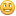And about SPK: I think having full, proper SPK support will do so much for the experience of just packaging and distributing games. Especially if we can make Sphere like a runtime and have good SPK support in the game launcher (like the startup game or an external launcher like Spherun).

• Fat Cerberus
••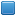• Global Moderator
• Sphere Developer
Re: Gravity Simulation
##### Reply #7 – December 20, 2013, 11:15:01 pm
If it were me, I would implement SPK support for backwards compatibility, but change the specification going forward to a proper archive format like .zip or .7z.  Would go a long way to making Sphere more modern, I think, and honestly, there's no real benefit to having a proprietary format just for packed Sphere games when it's ultimately just a glorified archive anyway.
Sphere 5.6.2 - neoSphere engine - Cell compiler - SSj debugger
•